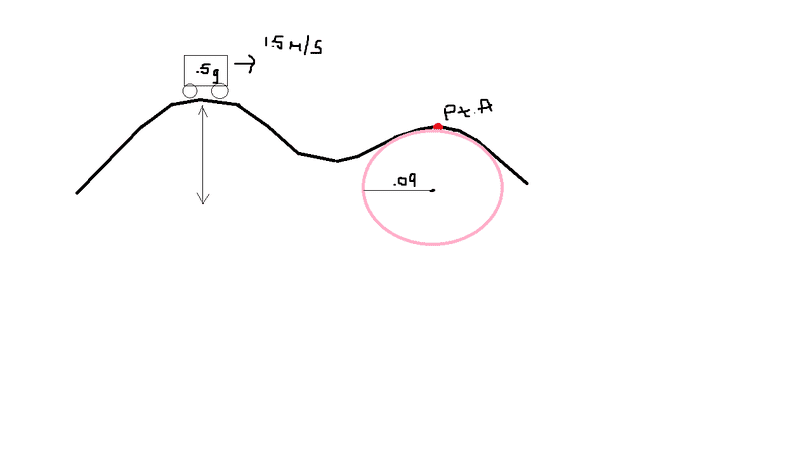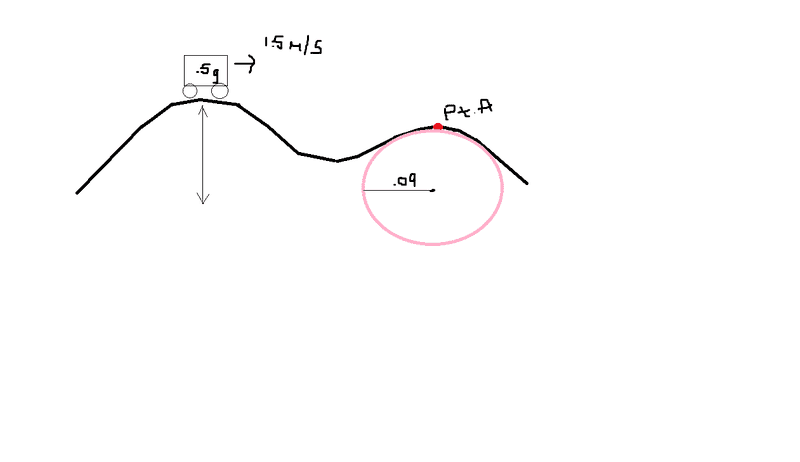# Physics problem: Radial acceleration, forces, and work & energy problem

• imisschem
So, if there isn't friction, the cart will continue moving at the speed it was going when it lost contact with the track.f

## Homework StatementIn case the numbers are hard to read:
Mass of the cart- .5g
Initial Velocity- 1.5 m/s
The height of the first hill is 2.

I need to find velocity (final, i think)
force(s) on the cart at point A
the magnitude of the force(s) at point a
Work Non Conservative if there was friction at point A
How would you change the set up if the car lost contact with point A?

## The Attempt at a Solution

So, I don't know.. I think I have an idea of how to start? This problem has me so lost.

a) To find the velocity, I tried doing
EFr=mar
EF=m(v^2/r)
and then, I wasn't quite sure of the radial forces.. I know that there is gravity obviously, but I didn't know if normal force canceled out because it was at the top? But I figured this equation was right because i have the mass and radius.

b) For the forces on the cart at point A.. again, I have gravity and normal force, but i don't know if force normal cancels out.

c) I think I know how to find the magnitude.. Fg=mg and Fn=mg? Fn could be wrong.

d) Work non conservative.. would be friction. So, Wnc=(coefficient of friction)(Fn)(displacement)

e) and i really don't know how to change the setup, because i don't really know what's going on at point a that would make the car lose contact with the track.

## Homework StatementIn case the numbers are hard to read:
Mass of the cart- .5g
Initial Velocity- 1.5 m/s
The height of the first hill is 2.

I need to find velocity (final, i think)
force(s) on the cart at point A
the magnitude of the force(s) at point a
Work Non Conservative if there was friction at point A
How would you change the set up if the car lost contact with point A?

## The Attempt at a Solution

So, I don't know.. I think I have an idea of how to start? This problem has me so lost.

a) To find the velocity, I tried doing
EFr=mar
EF=m(v^2/r)
and then, I wasn't quite sure of the radial forces.. I know that there is gravity obviously, but I didn't know if normal force canceled out because it was at the top? But I figured this equation was right because i have the mass and radius.

b) For the forces on the cart at point A.. again, I have gravity and normal force, but i don't know if force normal cancels out.

c) I think I know how to find the magnitude.. Fg=mg and Fn=mg? Fn could be wrong.

d) Work non conservative.. would be friction. So, Wnc=(coefficient of friction)(Fn)(displacement)

e) and i really don't know how to change the setup, because i don't really know what's going on at point a that would make the car lose contact with the track.

Firstly, was the mass of the cart really only half a gram?

The first hill has height 2.0 m, How high is the second hill?

The radius of the hill doesn't necessarily help - for example many "speed humps" in a street have a radius of several metres, but are only 15 - 20 cm high.

Also, that is a VERY sharp hill - with a radius of only 9 cm !

it could have been .5 kg, the diagram was so hard to read since it was a copy.
the height of the second one i believe was 1.9, but, those numbers are so close and the diagram seemed off.. i don't know. i just like.. even without the numbers i have no idea how to solve the problem other than what i did, which is most likely inaccurate.

it could have been .5 kg, the diagram was so hard to read since it was a copy.
the height of the second one i believe was 1.9, but, those numbers are so close and the diagram seemed off.. i don't know. i just like.. even without the numbers i have no idea how to solve the problem other than what i did, which is most likely inaccurate.

At the top of the first hill, the mass has KE [it is traveling at 1.5 m/s], and PE, [it is 2m up]

That will get a total energy, which will be maintained until frictoion comes ito it [I don'r see that it does]

At the top of the second hill, the object has less PE [it is only 1.9 m up] so more kinetic energy.
That will enable you to find the velocity over the hill.

hopefully you can proceed from there.

so.. I'm still confused.
do i need to get the total energy first (by finding PE and KE and adding them), then account for Wnc because of friction, and THEN get the velocity?

so.. I'm still confused.
do i need to get the total energy first (by finding PE and KE and adding them), then account for Wnc because of friction, and THEN get the velocity?

Do a calculation assuming there is no friction, and we can proceed from there.

Okay..

PE1 + KE1 = PE2 + KE2
mgh + 1/2mv^2 = mgh + 1/2mv^2
(.5kg)(9.8)(2) + .5(.5)(1.5)^2 = (.5)(9.8)(1.9) + .5(.5)v^2
9.8+ .5625 = 9.31 + .25v^2
v= 8.2?

nevermind, i got 2.05... calculator error

nevermind, i got 2.05... calculator error

Fine, DO you know how to proceed from there?

not really...

well actually for the most part yes. I'm just confused.. is there normal force at point A? because at the highest velocity i thought it canceled out. but i don't actually know.

not really...

back to the original question:

I need to find velocity (final, i think)
force(s) on the cart at point A
the magnitude of the force(s) at point a
Work Non Conservative if there was friction at point A
How would you change the set up if the car lost contact with point A?

Right you have the velocity - line 1

At point A we can hope that the forces are : weight [mg] down, and some reaction force up.

Together, they need to add to the Centripetal force needed to travel in a circle of radius 0.09 m. Fc is given by mv2/R
You know mass, velocity and radius so you can get that.
The weight force down is easy too - just under 5N.

Lets suppose that Fc was 2N down
That would be achieved by the track pushing up with a force of ~3N.
Weight down plus track up 5N down + 3N up = 2N down.

If this car is going too fast, the required down force may exceed the weight force. That means the cart will leave the track.
You would need to reduce Fc in that case - either reduce v or increase R [or both]

v would be reduced if there was friction, or could be reduced if the second hill was higher.
R could be increased by changing the shape of the track

well actually for the most part yes. I'm just confused.. is there normal force at point A? because at the highest velocity i thought it canceled out. but i don't actually know.

Just because you have a hill doesn't mean the normal force will be "cancelled out". You have to get it just right for it to be "cancelled".

The normal force will certainly be less that what it would have been if the track was flat, but how much less depends on the shape of the track and the speed of the cart.

Okay, that makes sense! Thank you very much!